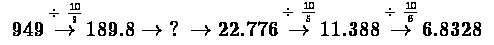## Number Series Practice Questions for IBPS, SBI and other bank exams

Number Series Practice Questions for IBPS, SBI and other bank exams
Directions (1 – 5): Find the wrong term in the following number series:
1. 7    37    91   167    271    397
A) 37
B) 91
C) 167
D) 271
E) 397
2. 8.1, 9.2, 17.3, 26.5, 43.8, 71.5, 114.1
A) 17.3
B) 26.5
C) 43.8
D) 9.2
E) None of these
3. 4015, 4395, 4140, 4272, 4265, 4145
A) 4272
B) 4395
C) 4265
D) 4145
E) 4015
4. 8    56    152   276    488    728
A) 56
B) 276
C) 152
D) 488
E) 728
5. 2   8   17   29   44   60   83   107
A) 60
B) 44
C) 29
D) 83
E) 17
Directions (6 – 10): What should come in place of the question mark (?) in the following number series?
6. 949        189.8    ?              22.776     11.388               6.8328
A) 48.24
B) 53.86
C) 74.26
D) 56.94
E) None of these
7. 5963       ?              5938       5913       5877       5828
A) 5932
B) 5940
C) 5951
D) 5954
E) None of these
8. 7              33           137         553         ?              8873
A) 1217
B) 2217
C) 2117
D) 2127
E) None of these
9. 88,       ?,          264,                 660,                 1980,              6930,              27720
A) 124
B) 120
C) 132
D) 224
E) 111
10. 1,         3,         10,       29,       74,       ?,          382
A) 173
B) 154
C) 189
D) 212
E) 222
Solutions:
1. C) The given series is in the pattern:
→ 7 = 23 – 13
→ 37 = 43 – 33
→ 91 = 63 – 53
→ 167 ≠ 83 – 73 = 169
→ 271 = 103 – 93
→ 397 = 123 – 113
So, the wrong term in the series is 167.
2. E) The pattern of the given series is:
→ 8.1,
→ 9.2,
→ 17.3 = 9.2 + 8.1,
→ 26.5 = 17.3 + 9.2,
→ 43.8 = 26.5 + 17.3,
Here the following number is the sum of previous two numbers
Next term should be:
→ 70.3 = 43.8 + 26.5,
Wrong term is 71.5
→ 114.1 = 70.3 + 43.8
3. A) The above series follows the pattern of alternate terms forming a series:
4015 + 125 = 4140
4395 – 125 = 4270
4140 + 125 = 4265
4270 – 125 = 4145
4140 + 125 = 4265
Hence, the wrong term is 4272.
4. B) The given series is in the pattern:
→ 8 = 23 – 03
→ 56 = 43 – 23
→ 152 = 63 – 43
→ 276 ≠ 83 – 63 = 296
→ 488 = 103 – 83
→ 728 = 123 – 103
So, the wrong term in the series is 276.
5. A) The given series is in following pattern:
2
2 + 3 × 2 = 8
8 + 3 × 3 = 17
17 + 3 × 4 = 29
29 + 3 × 5 = 44
44 + 3 × 6 = 62 ≠ 60
62 + 3 × 7 = 83
83 + 3 × 8 = 107
So, the wrong term is 60.
6. D) The pattern that the series is following can be shown easily in a diagram:Thus the unknown number is 189.8 ÷ 10/3 = 56.94
7. D) The given series is,
→ 5963,
→? = 5963 – 32 = 5954,
→ 5938 = 5954 – 42,
→ 5913 = 5938 – 52,
→ 5877 = 5913 – 62,
→ 5828 = 5877 - 72
? = 5954
8. B) The given series is,
→ 7,
→ 33 = 7 + 13 × 2,
→ 137 = 33 + 13 × 23,
→ 553 = 137 + 13 × 25,
→? = 553 + 13 × 27 = 2217,
→ 8873 = 2217 + 13 × 29
? = 2217
9. C) The pattern of the given number series is as following:
→ 88,
→ 88 × 1.5 = 132,
→ 132 × 2 = 264,
→ 264 × 2.5 = 660,
→ 660 × 3 = 1980,
→ 1980 × 3.5 = 6930,
→ 6930 × 4 = 27720
Hence, the required term in place of question mark in the given number series is 132.
10. A) The pattern of the given number series is as following-
→ 1
→ 1 × 2 + 12 = 3,
→ 3 × 2 + 22 = 10,
→ 10 × 2 + 32 = 29,
→ 29 × 2 + 42 = 74,
→ 74 × 2 + 52 = 173,
→ 173 × 2 + 62 = 382
Hence, the required number in the place of the question mark is 173.